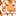Announcements
You can succeed best and quickest by helping others to succeed. Join the conversation.
cancel
Showing results for
Did you mean:Contributor III

## SPC Highlights

HI,

I have been trying to follow a very useful post from Erica Whalley at

QlikFit: Qlikview Lean tips #1: How to highlight series of points that violate control rules on a ch...

I have run into a problem though.

this expressions requires an average, which I want to be dynamic.

=if(RowNo()=1 or count(Key)>([Average]),0,above(R2CUM)+(count(Key)<=(Average]))

I have tried using the average expression

avg(aggr(Count(Key),Week))

but of course this just returns the count(Key) because it is averaging and aggregating it over the one week in the chart.

the only way around this I can find is to calculate the average manually as below

=if(RowNo()=1 or count(Key)>(count(total Key)/NoOfRows()),0,above(R2CUM)+(count(Key)<=(count(total Key)/NoOfRows())))

which is fine for highlighting points above/below the mean but I also want to highlight above or below the UCL and LCL.

so is there a way around this problem please?

thanks once again for your time.

Kind Regards

Steve

1 Solution

Accepted SolutionsMVP

You can calculate average like this

Avg(TOTAL Aggr(Count(Key), Week))

and stdev like this

Stdev(TOTAL Aggr(Count(Key), Week))

11 RepliesMaster III

Hi Steven,

ThanksMVP

Would you be able to share a sample where we can see the issue?Contributor III
Author

hi Sunny, sorry it has taken a while to respond - I have been off.

I can't see how to share the document?

SteveMVPContributor III
Author

Thanks Sunny - I was trying to reply through the inbox - hence the problem!

The formula in question is R2CUM. it works for highlighting points above and below the average but  I have had to manually calculate the average as you will see in the expression. The problem is I want to do the same thing for over the UCL or under the LCL - which I can't do from a manual expression -hence I have a problem...

Kind Regards

SteveMVP

Would you be able to expand what do you mean by manual expression?Contributor III
Author

The average is being calculated from

count(total Key)/NoOfRows()

rather than by using an expression from avg()

this means I cannot use stdev() to adapt the expression to highlight above and below UCL or LCL.MVP

You can calculate average like this

Avg(TOTAL Aggr(Count(Key), Week))

and stdev like this

Stdev(TOTAL Aggr(Count(Key), Week))Contributor III
Author

ahhh... is it the TOTAL that makes a difference? I tried it without the TOTAL and it returned count(Key)?

Brilliant - really appreciate your help - thank you.Community Browser